## portfolio optimization under higher moments (skewness-kurtosis)

Posts: 2
Hello dears,

knowing that the variance of portfolio is as follow: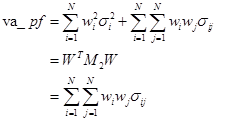I just set a program of minimization of portfolio variance where the objective function is as follow:

min f = (sum{i in N}(sum{j in N}(w[i]*var[i,j]*w[j])));

var[i,j] is the covariance between i and j.

and wi are decison variables (optimal weight)

Now, I 'm trying to maximize the skewness of portfolio

I calculated the matrix of skewness co-skewness of assets returns. But I can not express the objective function as it contains a triple sum. it is having the following forms.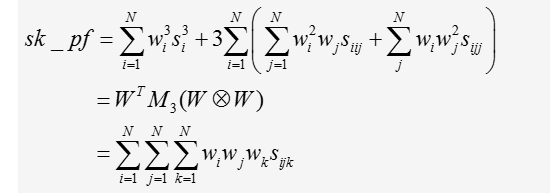Where wi are decision variables (vector of optimal weights to invest in each asset).

and sijk are assets (i,j,k)coskewness

W is the vector of weghts

M3 is the skewenss co-skewness matrix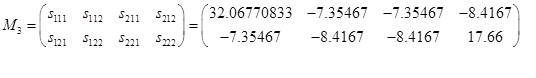1 ACCEPTED SOLUTION

Accepted Solutions

## Re: portfolio optimization under higher moments (skewness-kurtosis)

Yes, I understand. You asked how to write the objective function, which I provided. The function is vectorized and will support an arbitrary number of assets.  You just need to use the objective function as part of a constrained nonlinear optimization. See the SAS/IML documentation chapter about nonlinear optimization, and especially the "Getting Started" example that shows how to run a linearly constrained optimization.

I like the NLPNRA algorithm myself.  You can read about how to specify a linear constraint.

One change from before. I forgot that the NLP routines pass in a row vector as the parameter. Therefore you want to transpose the input argument before using it.  The following code gives the optimal value for your 2-D example. To support more variables, just update the new M3 matrix, the constraint matrix, and the initial guess.

``````proc iml;
M3 = {32 -7 -7 -8,
-7 -8 -8 17};

start skpf(p) global(M3);
w = p`;   /* w is column vector */
return w` * M3 * (w@w);
finish;

/* specify linear constraints  */
con = { 0  0  .   .,  /* min w[i]   */
1  1  .   .,  /* max w[i]   */
1  1  0   1}; /* sum(w) = 1 */
x0 = {0.5 0.5};
optn = {1    /* maximize objective function */
1 }; /* summarize iteration history */
call nlpnra(rc, xOpt, "skpf", x0, optn, con);
maxVal = skpf(xOpt);
print rc, xOpt maxVal;
``````
7 REPLIES 7

## Re: portfolio optimization under higher moments (skewness-kurtosis)

You can implement triple-nested DO loops if you want to implement the summation formula, but the second line gives the matrix formula, which will be much easier and faster to compute.

Assuming that the weight vector is a column vector, the objective function is the matrix product w`*M3*(w@w), where w@w is the Kronecker product:

``````proc iml;
M3 = {32 -7 -7 -8,
-7 -8 -8 17};

start skpf(w) global(M3);   /* w is column vector */
return w` * M3 * (w@w);
finish;

w = {0.2, 0.8};  /* w is column vector */
v = skpf(w);``````

I assume that the sum of the weights is unity. If so, then you need to include an constaint when you optimize because there is really only N-1 parameters:  w[n] = 1 - (w + w + ... + w[n-1]).

In particular, for your example where N=2, you get the following graph of the objective function. Notice that the maximum is on the boundary for this example: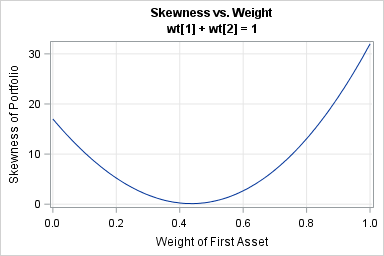## Re: portfolio optimization under higher moments (skewness-kurtosis)

Hi,

Thank you, as I understand your solution allows me to compute the skewness of  portfolio (wi (weights) are known).
However, in my case the wi are unknown and I try to find them (decision variables).
In fact I am trying to write the expression of the portfolio skewness (to maximize) as follow:
(for example for Two titles) we have: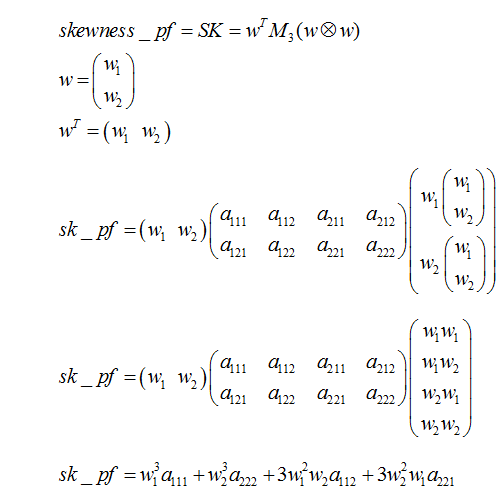For N assets, how could I write it ?

In fact, my objectiv function is as follow :

Max sk_pf

Thank you so much in advance!

## Re: portfolio optimization under higher moments (skewness-kurtosis)

Yes, I understand. You asked how to write the objective function, which I provided. The function is vectorized and will support an arbitrary number of assets.  You just need to use the objective function as part of a constrained nonlinear optimization. See the SAS/IML documentation chapter about nonlinear optimization, and especially the "Getting Started" example that shows how to run a linearly constrained optimization.

I like the NLPNRA algorithm myself.  You can read about how to specify a linear constraint.

One change from before. I forgot that the NLP routines pass in a row vector as the parameter. Therefore you want to transpose the input argument before using it.  The following code gives the optimal value for your 2-D example. To support more variables, just update the new M3 matrix, the constraint matrix, and the initial guess.

``````proc iml;
M3 = {32 -7 -7 -8,
-7 -8 -8 17};

start skpf(p) global(M3);
w = p`;   /* w is column vector */
return w` * M3 * (w@w);
finish;

/* specify linear constraints  */
con = { 0  0  .   .,  /* min w[i]   */
1  1  .   .,  /* max w[i]   */
1  1  0   1}; /* sum(w) = 1 */
x0 = {0.5 0.5};
optn = {1    /* maximize objective function */
1 }; /* summarize iteration history */
call nlpnra(rc, xOpt, "skpf", x0, optn, con);
maxVal = skpf(xOpt);
print rc, xOpt maxVal;
``````

## Re: portfolio optimization under higher moments (skewness-kurtosis)

Hello,

Thank you very much, but I'm sorry, I really don't understand why using proc iml if I don't have to compute the value of portfolio skewness ( since weights are unkown). In fact, I'd like to express my objective function in terms of weights (wi) and I think that proc optmodel could be usuful!

## Re: portfolio optimization under higher moments (skewness-kurtosis)

I think you are not understanding the objective function that I wrote.

The argument is a VECTOR of weights: w = [w1, w2, w3, ..., wk].  The '*' operator performs matrix multiplication. So in fact

w` * M3 * (w@w)

is exactly the formula that you wrote.  It is merely the matrix formulation, but it reduces to the same (i,jk) summation.

## Re: portfolio optimization under higher moments (skewness-kurtosis)

Hello, I did not immediately reply your answer because I have taken a long time to understand it! It is so awesome! Thank you very much! I really appreciate your help! I read chapter 14 about Nonlinear optimization examples! I just discovered that SAS could do it!It’s so great!Nevertheless , I have one more problem. I have one linear constraint and one Nonlinear constraint (quartic constraint about portfolio kurtosis which is less than a scalar). As I understand I must use NLPQN instead of NLPNRA! IS that sufficient?

In the other hand, I still think about the mining of “optn”!

Finally, I want to state decision variables wi as positive, I question whether it been subject to a further constraint.

Sorry for those lots of questions. Thank you in advance!From The DO Loop